### Cross Validation

Deciding when to stop a selection method is a crucial issue in performing effect selection. Predictive performance of candidate models on data not used in fitting the model is one approach supported by PROC GLMSELECT for addressing this problem (see the section Using Validation and Test Data). However, in some cases, you might not have sufficient data to create a sizable training set and a validation set that represent the predictive population well. In these cases, cross validation is an attractive alternative for estimating prediction error.

In k-fold cross validation, the data are split into k roughly equal-sized parts. One of these parts is held out for validation, and the model is fit on the remaining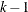parts. This fitted model is used to compute the predicted residual sum of squares on the omitted part, and this process is repeated for each of k parts. The sum of the k predicted residual sum of squares so obtained is the estimate of the prediction error that is denoted by CVPRESS. Note that computing the CVPRESS statistic for k-fold cross validation requires fitting k different models, and so the work and memory requirements increase linearly with the number of cross validation folds.

You can use the CVMETHOD= option in the MODEL statement to specify the method for splitting the data into k parts. CVMETHOD=BLOCK(k) requests that the k parts be made of blocks of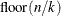or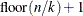successive observations, where n is the number of observations. CVMETHOD=SPLIT(k) requests that parts consist of observations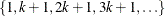,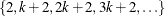, . . . ,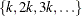. CVMETHOD=RANDOM(k) partitions the data into random subsets each with roughlyobservations. Finally, you can use the formatted value of an input data set variable to define the parts by specifying CVMETHOD=variable. This last partitioning method is useful in cases where you need to exercise extra control over how the data are partitioned by taking into account factors such as important but rare observations that you want to spread out across the various parts.

You can request details of the CVPRESS computations by specifying the CVDETAILS= option in the MODEL statement. When you use cross validation, the output data set created with an OUTPUT statement contains an integer-valued variable, _CVINDEX_, whose values indicate the subset to which an observation is assigned.

The widely used special case of n-fold cross validation when you have n observations is known as leave-one-out cross validation. In this case, each omitted part consists of one observation, and CVPRESS statistic can be efficiently obtained without refitting the model n times. In this case, the CVPRESS statistic is denoted simply by PRESS and is given by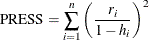where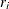is the residual and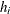is the leverage of the ith observation. You can request leave-one-out cross validation by specifying PRESS instead of CV with the options SELECT=, CHOOSE=, and STOP= in the MODEL statement. For example, if the number of observations in the data set is 100, then the following two PROC GLMSELECT steps are mathematically equivalent, but the second step is computed much more efficiently:

proc glmselect;
model y=x1-x10/selection=forward(stop=CV) cvMethod=split(100);
run;

proc glmselect;
model y=x1-x10/selection=forward(stop=PRESS);
run;


Hastie, Tibshirani, and Friedman (2001) include a discussion about choosing the cross validation fold. They note that as an estimator of true prediction error, cross validation tends to have decreasing bias but increasing variance as the number of folds increases. They recommend five- or tenfold cross validation as a good compromise. By default, PROC GLMSELECT uses CVMETHOD=RANDOM(5) for cross validation.

#### Using Cross Validation as the STOP= Criterion

You request cross validation as the stopping criterion by specifying the STOP=CV suboption of the SELECTION= option in the MODEL statement. At step k of the selection process, the best candidate effect to enter or leave the current model is determined. Note that here best candidate means the effect that gives the best value of the SELECT= criterion that need not be the CV criterion. The CVPRESS score for the model with this candidate effect added or removed is determined. If this CVPRESS score is greater than the CVPRESS score for the model at step k, then the selection process terminates at step k.

#### Using Cross Validation as the CHOOSE= Criterion

When you specify the CHOOSE=CV suboption of the SELECTION= option in the MODEL statement, the CVPRESS score is computed for the models at each step of the selection process. The model at the first step yielding the smallest CVPRESS score is selected.

#### Using Cross Validation as the SELECT= Criterion

You request cross validation as the selection criterion by specifying the SELECT=CV suboption of the SELECTION= option in the MODEL statement. At step k of the selection process, the CVPRESS score is computed for each model where a candidate for entry is added or a candidate for removal is dropped. The selected candidate for entry or removal is the one that yields a model with the minimal CVPRESS score. Note that at each step of the selection process, this requires forming the CVPRESS statistic for all possible candidate models at the next step. Since forming the CVPRESS statistic for k-fold requires fitting k models, using cross validation as the selection criterion is computationally very demanding compared to using other selection criteria.